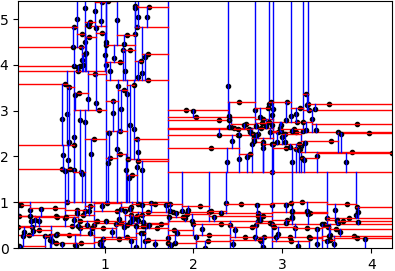pyclustering  0.10.1 pyclustring is a Python, C++ data mining library.
pyclustering.container.kdtree.kdtree_balanced Class Reference

Represents balanced static KD-tree that does not provide services to add and remove nodes after initialization. More...Inheritance diagram for pyclustering.container.kdtree.kdtree_balanced:

## Public Member Functions

Initializes balanced static KD-tree. More...

def __len__ (self)
Returns amount of nodes in the KD-tree. More...

def get_root (self)
Returns root of the tree. More...

Find node with specified coordinates and payload. More...

def find_node (self, point, cur_node=None)
Find node with coordinates that are defined by specified point. More...

def find_nearest_dist_node (self, point, distance, retdistance=False)
Find nearest neighbor in area with radius = distance. More...

def find_nearest_dist_nodes (self, point, distance)
Find neighbors that are located in area that is covered by specified distance. More...

## Detailed Description

Represents balanced static KD-tree that does not provide services to add and remove nodes after initialization.

In the term KD tree, k denotes the dimensionality of the space being represented. Each data point is represented as a node in the k-d tree in the form of a record of type node.

There is an example how to create KD-tree:

from pyclustering.container.kdtree import kdtree_balanced, kdtree_visualizer
from pyclustering.samples.definitions import FCPS_SAMPLES
tree_instance = kdtree_balanced(sample)
kdtree_visualizer(tree_instance).visualize()

Output result of the example above - figure 1.Fig. 1. Balanced KD-tree for sample 'Lsun'.
kdtree

Definition at line 243 of file kdtree.py.

## ◆ __init__()

 def pyclustering.container.kdtree.kdtree_balanced.__init__ ( self, points, payloads = None )

Initializes balanced static KD-tree.

Parameters
 [in] points (array_like): Points that should be used to build KD-tree. [in] payloads (array_like): Payload of each point in points.

Reimplemented in pyclustering.container.kdtree.kdtree.

Definition at line 269 of file kdtree.py.

## ◆ __len__()

 def pyclustering.container.kdtree.kdtree_balanced.__len__ ( self )

Returns amount of nodes in the KD-tree.

Returns
(uint) Amount of nodes in the KD-tree.

Definition at line 299 of file kdtree.py.

## ◆ find_nearest_dist_node()

 def pyclustering.container.kdtree.kdtree_balanced.find_nearest_dist_node ( self, point, distance, retdistance = False )

Find nearest neighbor in area with radius = distance.

Parameters
 [in] point (list): Maximum distance where neighbors are searched. [in] distance (double): Maximum distance where neighbors are searched. [in] retdistance (bool): If True - returns neighbors with distances to them, otherwise only neighbors is returned.
Returns
(node|list) Nearest neighbor if 'retdistance' is False and list with two elements [node, distance] if 'retdistance' is True, where the first element is pointer to node and the second element is distance to it.

Definition at line 431 of file kdtree.py.

## ◆ find_nearest_dist_nodes()

 def pyclustering.container.kdtree.kdtree_balanced.find_nearest_dist_nodes ( self, point, distance )

Find neighbors that are located in area that is covered by specified distance.

Parameters
 [in] point (list): Coordinates that is considered as centroid for searching. [in] distance (double): Distance from the center where searching is performed.
Returns
(list) Neighbors in area that is specified by point (center) and distance (radius).

Definition at line 458 of file kdtree.py.

## ◆ find_node()

 def pyclustering.container.kdtree.kdtree_balanced.find_node ( self, point, cur_node = None )

Find node with coordinates that are defined by specified point.

If node with specified parameters does not exist then None will be returned, otherwise required node will be returned.

Parameters
 [in] point (list): Coordinates of the point whose node should be found. [in] cur_node (node): Node from which search should be started.
Returns
(node) Node if it satisfies to input parameters, otherwise it return None.

Definition at line 415 of file kdtree.py.

Referenced by pyclustering.container.kdtree.kdtree.remove().

 def pyclustering.container.kdtree.kdtree_balanced.find_node_with_payload ( self, point, point_payload, cur_node = None )

Find node with specified coordinates and payload.

If node with specified parameters does not exist then None will be returned, otherwise required node will be returned.

Parameters
 [in] point (list): Coordinates of the point whose node should be found. [in] point_payload (any): Payload of the node that is searched in the tree. [in] cur_node (node): Node from which search should be started.
Returns
(node) Node if it satisfies to input parameters, otherwise it return None.

Definition at line 397 of file kdtree.py.

Referenced by pyclustering.container.kdtree.kdtree.remove().

## ◆ get_root()

 def pyclustering.container.kdtree.kdtree_balanced.get_root ( self )

Returns root of the tree.

Returns
(node) The root of the tree.

Definition at line 308 of file kdtree.py.

The documentation for this class was generated from the following file:
pyclustering.container.kdtree
Data Structure: KD-Tree.
Definition: kdtree.py:1
pyclustering.utils
Utils that are used by modules of pyclustering.
Definition: __init__.py:1